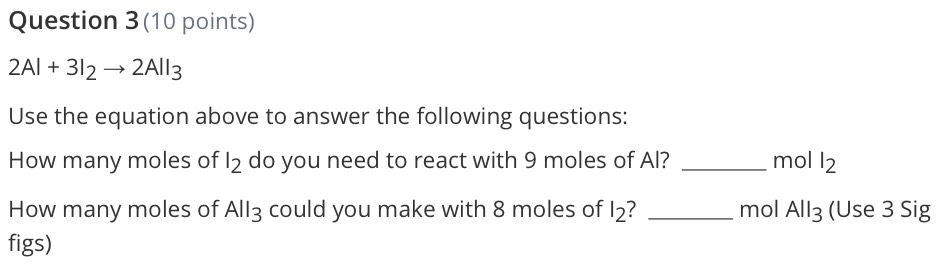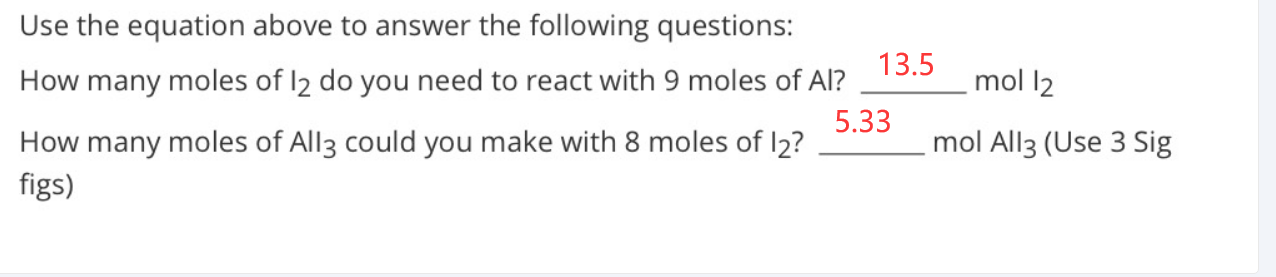### Still have math questions?

Arithmetic
Question$$2 Al + 3 l _ { 2 } \rightarrow 2 Al _ { 3 }$$

Use the equation above to answer the following questions: How many moles of $$I _ { 2 }$$ do you need to react with $$9$$ moles of $$Al$$ ? How many moles of $$All _ { 3 }$$ could you make with $$8$$ moles of $$I _ { 2 }$$ ? $$mol I _ { 2 }$$

figs)